Description of Programs

### Subdivision programs

We describe and illustrate here three programs, subdivide.c, tilepack.c, and squarect.c, which are written to run on unix platforms and whose source codes are available from this web site. All three programs were written by J. W. Cannon, W. J. Floyd, and W. R. Parry.

The first program, subdivide.c, implements one subdivision of a tiling of a quadrilateral, with respect to a finite subdivision rule. For details about finite subdivision rules, see the paper Finite subdivision rules. The program prompts you for a rules file and a tiling file for a quadrilateral, and returns the tiling file for the subdivision.

For example, if you enter the rules file pent.r and the tiling file pent.l0, then it will write the file pent.l1 after prompting you for the name. One could then reuse the program to subdivide pent.l1.

Here is the rules file pent.r.

```Number_of_tile-types_(not_counting_the_four_standard_end_types):
1
Size_(i.e.,_number_of_edges)_of_each_of_these_types:
5
Subdivision-tiling:
Type_number_(the_first_one_should_be_4_since_the_ends_are_typed_0_1_2_3):
4
Number_of_tiles_into_which_that_tile_type_is_subdivided:
6
Type_of_each_of_these_tiles_in_the_subdivision:
4 4 4 4 4 4
0 -1 -1 1 4 5 0 4 0 -1 -1
1 -1 -1 -1 -1 2 0 5 1 0 1
2 1 2 -1 -1 -1 -1 3 1 5 2
3 5 3 2 3 -1 -1 -1 -1 4 2
4 0 3 5 4 3 4 -1 -1 -1 -1
5 0 2 1 3 2 4 3 0 4 1
Corresponding_boundary-tiling:
Boundary_of_type_number_(the_first_should_be_4):
4
Number_of_edges_before_subdivision:
5
Number_of_edges_into_which_each_of_these_original_edges_is_subdivided:
2 2 2 2 2
Original_edgeid_-_numbers_in_pairs_for_subdivision_edges_(tileno_edgeno):
0  1 0 0 0
1  2 1 1 1
2  3 2 2 2
3  4 3 3 3
4  0 4 4 4
```

The lines with text are read as strings and discarded. This program was originally designed for computing combinatorial moduli of quadrilaterals. Because of this, there are four types, 0, 1, 2, and 3, corresponding to the bottom, left side, top, and right side of the quadrilateral. These are called end types. The rules file does not contain any information about the end types. For each of the other types, the rules file gives the size (number of edges), subdivision-tiling, and boundary-tiling.

In this example, the pentagonal subdivision rule, there is only one non-end type, a pentagon. This pentagon is subdivided into six tiles, which are all pentagons (type 4) since this is the only non-end type. The original pentagon and each of the pentagons in the subdivision has edges labeled 0,1,2,3,4 in the clockwise orientation, as shown in the following figure.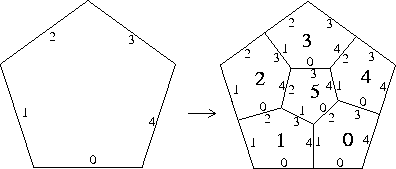The next information in the rules file gives the subdivided tiles across from the edges of each subdivided tile. If an edge is on the boundary, -1 is given for the label of the subdivided tile.

Finally, the rules file gives boundary information. The pentagon has 5 edges, and each is subdivided into two pieces. For each edge the file gives the tile numbers and edge numbers of the subtiles across from each subedge, reading clockwise from the outside of the pentagon.

The tiling files gives similar data for the tiling of a quadrilateral by tiles of the types given in the rules files. For example, here is pent.l0.

```Number_of_tiles_including_the_four_standard_ends:
5
Type_of_each_of_these_tiles:
0 1 2 3 4
Size_of_the_tiles
1 1 2 1 5
0 4 0
1 4 1
2 4 3 4 2
3 4 4
4 0 0 1 0 2 1 2 0 3 0
```
The quadrilateral has only one tile, a pentagon (of type 4, since that is the only non-end type. But there are still the special end types 0 (the bottom), 1 (the left side), 2 (the top), and 3 (the right side). One of the vertices of the pentgaon is the midpoint of the top, and each of the other vertices is a vertex of the quadrilateral. Type 0, the bottom, has one edge, and across from it is tile 4. Type 1, the left side, has one edge, and across from it is tile 4. Type 2, the top, has two edges, and across from each is tile 4. Type 3, the right side, has one edge, and across from it is tile 4. Edge 0 of tile 4 is on the bottom, and we read what is across from the edges of tile 4 in the clockwise direction. What we see across from the edges is 0 (bottom), 1 (left side), 2 (top), 2(top), and 3 (right side). We choose the name pent.l0 because we are thinking of this as level 0 in the family of subdivisions of the pentagon.

The second program, tilepack.c, inputs a tiling file and outputs a script file with the appropriate data for using Ken Stephenson's circle packing program, CirclePack, to draw the tiling. CirclePack is available from http://www.math.utk.edu/~kens. The program prompts you for the name of the tiling file and the name of the script file. It also asks you whether you want the geometry of the circle packing to be Euclidean or hyperbolic. For example, you could input the tiling file pent.l2 (the second subdivision of pent.l0), and output the file pentl2.xmd.

After tile pack reads in the tiling file T, it creates (but does not write) a tiling file for the triangulation K obtained by adding a vertex for the barycenter of each face and joining it by an edge to each vertex of the face. CirclePack will then find a circle for each vertex so that two circles are tangent exactly if the vertices bound an edge and are disjoint otherwise.

Here are the commands from the script lines at the beginning of pentl2.xmd.

```act 0;infile_read pentl2.xmd.p;
repack;layout;disp -w -c;geom_to_e;
set_aim 1.0 b;set_aim 0.5 37 41 45 53
gamma 49;repack;fix; disp -w -c -e b;
mark -cw -c a(37,101);disp -w -e m;
```
The first command designates 0 as the active pack and reads in the data from the file. (The default in CirclePack is that there are three packs, 0, 1, and 2, and one can work with three different packings at the same time.) The second command packs the packing (processes through the algorithm to compute the radii of the circles), fixes the packing (to find the centers of the circles), and displays the circles on the screen. This command may need to be repeated if there are a lot (e.g., more than 10,000) of vertices. The next command sets the geometry to Euclidean, sets the aim (angle sum) of each vertex on the boundary to be 1.0 (multplied by pi), and then sets the aims of the four corners of the quadrilateral to be 0.5 (pi/2). This means that you will be packing a Euclidean rectangle.

Tilepack numbers the vertices as follows. It first numbers the vertices (1-36 in this case) that are barycenters, then the vertices (37-40) on the bottom except for the lower right corner, then the vertices (41-44) on the left but not the lower left corner, then the vertices (45-52) on the top but not the upper left corner, then the vertices (53-56) on the right side but not the upper right corner, and then the interior vertices that are not barycenters.

The next command sets vertex 49 (the midpoint of the top) as the gamma vertex. (The gamma vertex has center on the positive y-axis.) If you can see what it is, it it convenient to designate the alpha vertex. (The alpha vertex has center at the origin; you can get the number of a vertex by clicking on its circle.) It then repacks and refixes the packings, and displays the circles and the edges on the boundary.

The next line marks the vertices that are not barycenters, and displays all of the edges between marked vertices. This is how you get the drawing of the tiling that you started with.

Here is the output of running pentl2.cmd.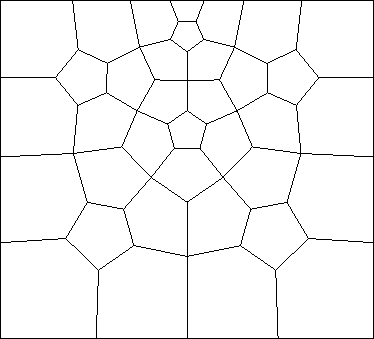Recall that this is a tiling of the second subdivision of a pentagon, which has been constrained to pack a quadrilateral. You can easily adapt the program to get a tiling of a pentagon instead of a rectangle. Change the third line of the script file to the following.

```[]:= geom_to_e;set_aim 1.0 b;set_aim 0.6 37 41 45 49 53
```

This set the aims at the boundary to be 1 (pi), and then sets the aims of the five corners of the pentagon to be .6 (3 pi/5). It was easy to do this because we chose the fifth vertex of the pentagon to be the midpoint of the top. Here is the output of this modification of pentl2.cmd.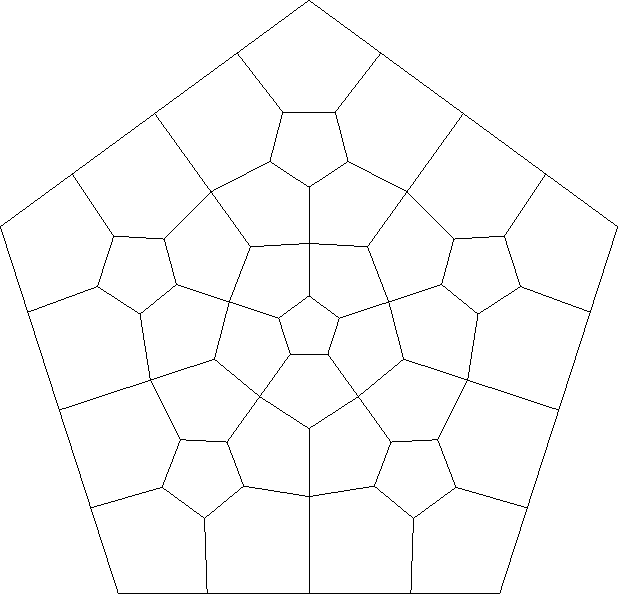We now do an example, the dodecahedral variant, with three non-end types. Type 4 is a pentagon, type 5 is a quadrilateral, and type 6 is a triangle. (There is no need for different types to have different numbers of edges.) Here is the rules file dvar.r.
```   Number_of_tile-types_(not_counting_the_four_standard_end_types):
3
Size_(i.e.,_number_of_edges)_of_each_of_these_types:
5 4 3
Subdivision-tiling:
Type_number_(the_first_one_should_be_4_since_the_ends_are_typed_0_1_2_3):
4
Number_of_tiles_into_which_that_tile_type_is_subdivided:
11
Type_of_each_of_these_tiles_in_the_subdivision:
4 6 4 6 4 4 6 4 6 4 6
0 -1 -1 -1 -1 6 0 5 1 1 4
1 -1 -1 0 4 2 1
2 -1 -1 1 2 5 0 3 1 -1 -1
3 -1 -1 2 3 4 1
4 -1 -1 3 2 5 4 10 0 -1 -1
5 2 2 0 3 7 0 9 0 4 2
6 0 2 -1 -1 7 1
7 5 2 6 2 -1 -1 -1 -1 8 0
8 7 4 -1 -1 9 2
9 5 3 8 2 -1 -1 -1 -1 10 1
10 4 3 9 4 -1 -1
Corresponding_boundary-tiling:
Boundary_of_type_number_(the_first_should_be_4):
4
Number_of_edges_before_subdivision:
5
Number_of_edges_into_which_each_of_these_original_edges_is_subdivided:
3 3 3 3 3
Original_edgeid_-_numbers_in_pairs_for_subdivision_edges_(tileno_edgeno):
0 0 0 1 0 2 0
1 7 2 6 1 0 1
2 9 2 8 1 7 3
3 4 4 10 2 9 3
4 2 4 3 0 4 0
Subdivision-tiling:
Type_number_(the_first_one_should_be_4_since_the_ends_are_typed_0_1_2_3):
5
Number_of_tiles_into_which_that_tile_type_is_subdivided:
9
Type_of_each_of_these_tiles_in_the_subdivision:
4 6 4 6 5 6 4 6 4
0 -1 -1 -1 -1 3 0 4 0 1 1
1 -1 -1 4 1 2 1
2 -1 -1 1 3 5 0 -1 -1
3 0 2 -1 -1 6 0 4 1
4 1 2 3 3 5 1
5 2 2 4 2 6 3 -1 -1
6 3 2 -1 -1 -1 -1 5 2
7 6 3 -1 -1 8 1
8 4 2 7 2 -1 -1 -1 -1
Corresponding_boundary-tiling:
Boundary_of_type_number_(the_first_should_be_4):
5
Number_of_edges_before_subdivision:
4
Number_of_edges_into_which_each_of_these_original_edges_is_subdivided:
3 3 3 3
Original_edgeid_-_numbers_in_pairs_for_subdivision_edges_(tileno_edgeno):
0 0 0 1 0 2 0
1 6 1 3 1 0 1
2 8 2 7 1 6 2
3 2 4 5 2 8 3
Subdivision-tiling:
Type_number_(the_first_one_should_be_4_since_the_ends_are_typed_0_1_2_3):
6
Number_of_tiles_into_which_that_tile_type_is_subdivided:
7
Type_of_each_of_these_tiles_in_the_subdivision:
5 5 5 5 6 5 5
0 -1 -1 -1 -1 3 0 1 1
1 -1 -1 0 3 4 0 2 1
2 -1 -1 1 3 5 0 -1 -1
3 0 2 -1 -1 6 0 4 1
4 1 2 3 3 5 1
5 2 2 4 2 6 3 -1 -1
6 3 2 -1 -1 -1 -1 5 2
Corresponding_boundary-tiling:
Boundary_of_type_number_(the_first_should_be_4):
6
Number_of_edges_before_subdivision:
3
Number_of_edges_into_which_each_of_these_original_edges_is_subdivided:
3 3 3
Original_edgeid_-_numbers_in_pairs_for_subdivision_edges_(tileno_edgeno):
0 0 0 1 0 2 0
1 6 1 3 1 0 1
2 2 3 5 3 6 2
```

The rules file is similar to pent.r, except that now the type information in non-trivial. You first get the data for the pentagon (type 4), then for the quadrilateral (type 5), and finally for the triangle (type 6). The numbering of the edges of the subtiles is shown below.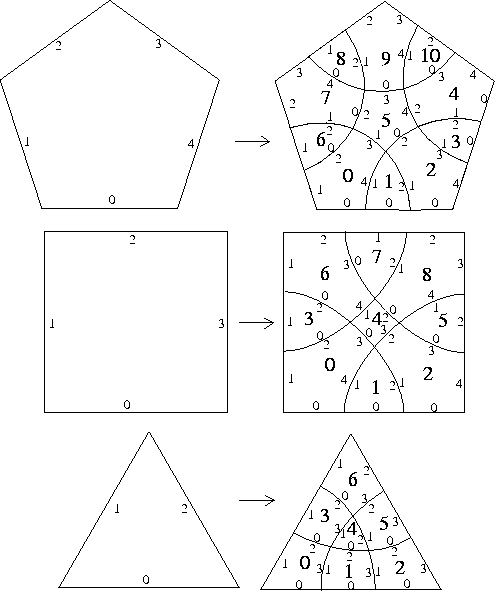Here is the tiling file dvarq.l0 for the level 0 file of a quadrilateral tiled by one tile of type 5.

```Number_of_tiles_including_the_four_standard_ends:
5
Type_of_each_of_these_tiles:
0 1 2 3 5
Size_of_the_tiles:
1 1 1 1 4
0 4 0
1 4 1
2 4 3 4 2
3 4 4
4 0 0 1 0 2 1 2 0 3 0
```
And here is the tiling file dvarp.l0 for the level 0 file for a quarilateral with a single tile of type 4 (and with two edges of the pengaton on the top of the quadrilateral).
```Number_of_tiles_including_the_four_standard_ends:
5
Type_of_each_of_these_tiles:
0 1 2 3 4
Size_of_the_tiles:
1 1 2 1 5
0 4 0
1 4 1
2 4 3 4 2
3 4 4
4 0 0 1 0 2 1 2 0 3 0
```

The third program, squarect.c, inputs the tiling file for a quadrilateral and outputs a postscript file for the associated squared rectangle. The squaring of the rectangle is done using the cyclic algorithm, as described in Squaring rectangles: the finite Riemann mapping theorem, by J.W. Cannon, W.J. Floyd, and W.R. Parry, Contemporary Mathematics, Volume 169, 1994.

The next figure shows the result of running squarect.c with the input file pent.l2.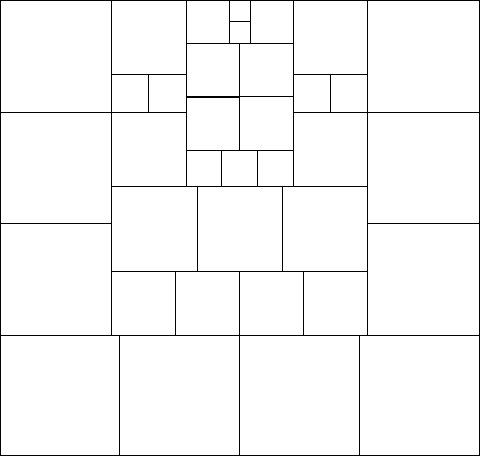Here are some other rules and tiling files for examples.

The rules file dodec.r and the tiling files dodecq.l0 and dodecp.l0 for the dodecahedral subdivision rule.

The rules file rtvar.r and the tiling file rtvar.l0 for the right triangle variant subdivision rule.

The rules file twpent.r and the tiling file twpent.l0 for the twisted pentagonal subdivision rule.